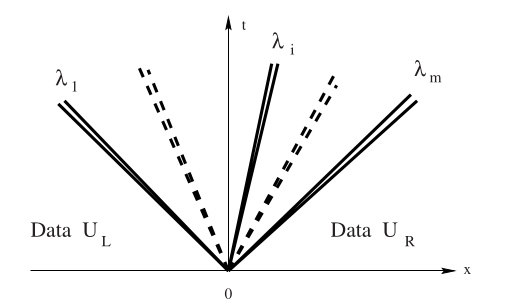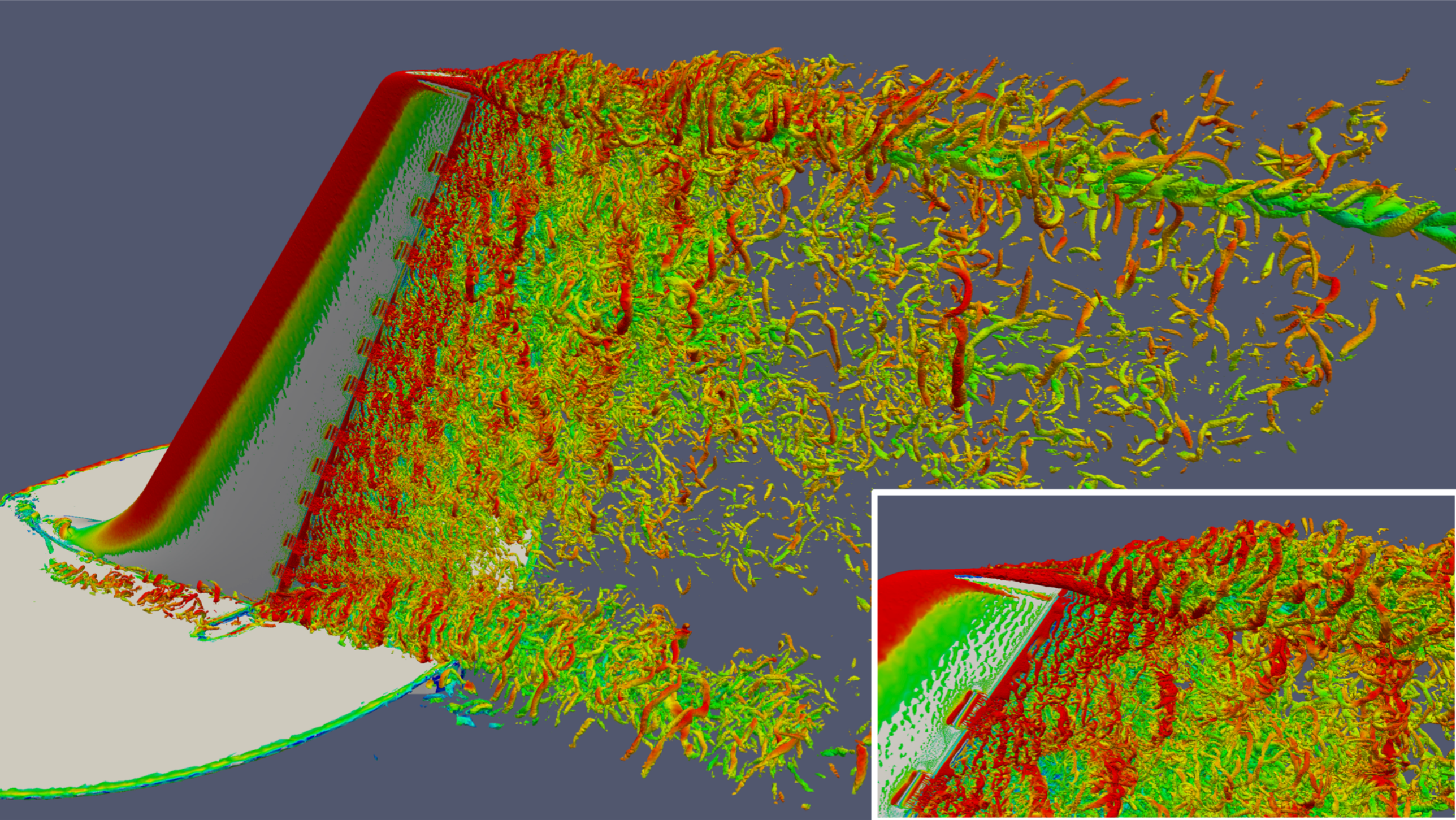# 2022-01-24 Convergence classes¶

• Office hours: Monday 9-10pm, Tuesday 2-3pm, Thursday 2-3pm

• This week will stay virtual. Plan is to start in-person the following Monday (Jan 31)

## Last time¶

• Forward and backward error

• Computing volume of a polygon

• Rootfinding examples

• Use Roots.jl to solve

• Introduce Bisection

## Today¶

• Discuss rootfinding as a modeling tool

• Limitations of bisection

• Convergence classes

• Intro to Newton methods

using Plots
default(linewidth=4)


# Stability demo: Volume of a polygon¶

X = ([1 0; 2 1; 1 3; 0 1; -1 1.5; -2 -1; .5 -2; 1 0])
R(θ) = [cos(θ) -sin(θ); sin(θ) cos(θ)]
Y = X * R(deg2rad(10))' .+ [1e4 1e4]
plot(Y[:,1], Y[:,2], seriestype=:shape, aspect_ratio=:equal)using LinearAlgebra
function pvolume(X)
n = size(X, 1)
vol = sum(det(X[i:i+1, :]) / 2 for i in 1:n-1)
end

@show pvolume(Y)
[det(Y[i:i+1, :]) for i in 1:size(Y, 1)-1]
A = Y[2:3, :]
sum([A[1,1] * A[2,2], - A[1,2] * A[2,1]])
X

pvolume(Y) = 9.249999989226126

8×2 Matrix{Float64}:
1.0   0.0
2.0   1.0
1.0   3.0
0.0   1.0
-1.0   1.5
-2.0  -1.0
0.5  -2.0
1.0   0.0

• Why don’t we even get the first digit correct? These numbers are only $$10^8$$ and $$\epsilon_{\text{machine}} \approx 10^{-16}$$ so shouldn’t we get about 8 digits correct?

# Rootfinding¶

Given $$f(x)$$, find $$x$$ such that $$f(x) = 0$$.

We’ll work with scalars ($$f$$ and $$x$$ are just numbers) for now, and revisit later when they vector-valued.

## Change inputs to outputs¶

• $$f(x; b) = x^2 - b$$

• $$x(b) = \sqrt{b}$$

• $$f(x; b) = \tan x - b$$

• $$x(b) = \arctan b$$

• $$f(x) = \cos x + x - b$$

• $$x(b) = ?$$

We aren’t given $$f(x)$$, but rather an algorithm f(x) that approximates it.

• Sometimes we get extra information, like fp(x) that approximates $$f'(x)$$

• If we have source code for f(x), maybe it can be transformed “automatically”

# Nesting matryoshka dolls of rootfinding¶

• I have a function (pressure, temperature) $$\mapsto$$ (density, energy)

• I need (density, energy) $$\mapsto$$ (pressure, temperature)

• I know what condition is satisfied across waves, but not the state on each side.• Given a proposed state, I can measure how much (mass, momentum, energy) is not conserved.

• Find the solution that conserves exactly

• I can compute lift given speed and angle of attack

• How much can the plane lift?

• How much can it lift on a short runway?# Discuss: rootfinding and inverse problems¶

• Inverse kinematics for robotics

• (statics) how much does each joint an robotic arm need to move to grasp an object

• (with momentum) fastest way to get there (motors and arms have finite strength)

• similar for virtual reality

• Infer a light source (or lenses/mirrors) from an iluminated scene

• Imaging

• radiologist seeing an x-ray is mentally inferring 3D geometry from 2D image

• computed tomography (CT) reconstructs 3D model from multiple 2D x-ray images

• seismology infers subsurface/deep earth structure from seismograpms

# Iterative bisection¶

f(x) = cos(x) - x
hasroot(f, a, b) = f(a) * f(b) < 0
function bisect_iter(f, a, b, tol)
hist = Float64[]
while abs(b - a) > tol
mid = (a + b) / 2
push!(hist, mid)
if hasroot(f, a, mid)
b = mid
else
a = mid
end
end
hist
end

bisect_iter (generic function with 1 method)

length(bisect_iter(f, -1, 3, 1e-20))

56


# Let’s plot the error¶

$\lvert \texttt{bisect}^k(f, a, b) - r \rvert, \quad k = 1, 2, \dotsc$

where $$r$$ is the true root, $$f(r) = 0$$.

hist = bisect_iter(f, -1, 3, 1e-10)
r = hist[end] # What are we trusting?
hist = hist[1:end-1]
scatter( abs.(hist .- r), yscale=:log10)
ks = 1:length(hist)
plot!(ks, 4 * (.5 .^ ks))Evidently the error $$e_k = x_k - x_*$$ after $$k$$ bisections satisfies the bound

$|e^k| \le c 2^{-k} .$

# Convergence classes¶

A convergent rootfinding algorithm produces a sequence of approximations $$x_k$$ such that

$\lim_{k \to \infty} x_k \to x_*$
where $$f(x_*) = 0$$. For analysis, it is convenient to define the errors $$e_k = x_k - x_*$$. We say that an iterative algorithm is $$q$$-linearly convergent if
$\lim_{k \to \infty} |e_{k+1}| / |e_k| = \rho < 1.$
(The $$q$$ is for “quotient”.) A smaller convergence factor $$\rho$$ represents faster convergence. A slightly weaker condition ($$r$$-linear convergence or just linear convergence) is that
$|e_k| \le \epsilon_k$
for all sufficiently large $$k$$ when the sequence $$\{\epsilon_k\}$$ converges $$q$$-linearly to 0.

ρ = 0.8
errors = [1.]
for i in 1:30
next_e = errors[end] * ρ
push!(errors, next_e)
end
plot(errors, yscale=:log10, ylims=(1e-10, 1))e = hist .- r
scatter(abs.(errors[2:end] ./ errors[1:end-1]), ylims=(0,1))# Remarks on bisection¶

• Specifying an interval is often inconvenient

• An interval in which the function changes sign guarantees convergence (robustness)

• No derivative information is required

• If bisection works for $$f(x)$$, then it works and gives the same accuracy for $$f(x) \sigma(x)$$ where $$\sigma(x) > 0$$.

• Roots of even degree are problematic

• A bound on the solution error is directly available

• The convergence rate is modest – one iteration per bit of accuracy

# Newton-Raphson Method¶

Much of numerical analysis reduces to Taylor series, the approximation

$f(x) = f(x_0) + f'(x_0) (x-x_0) + f''(x_0) (x - x_0)^2 / 2 + \underbrace{\dotsb}_{O((x-x_0)^3)}$
centered on some reference point $$x_0$$.

In numerical computation, it is exceedingly rare to look beyond the first-order approximation

$\tilde f_{x_0}(x) = f(x_0) + f'(x_0)(x - x_0) .$
Since $$\tilde f_{x_0}(x)$$ is a linear function, we can explicitly compute the unique solution of $$\tilde f_{x_0}(x) = 0$$ as
$x = x_0 - f(x_0) / f'(x_0) .$
This is Newton’s Method (aka Newton-Raphson or Newton-Raphson-Simpson) for finding the roots of differentiable functions.

# An implementation¶

function newton(f, fp, x0; tol=1e-8, verbose=false)
x = x0
for k in 1:100 # max number of iterations
fx = f(x)
fpx = fp(x)
if verbose
println("[$k] x=$x  f(x)=$fx f'(x)=$fpx")
end
if abs(fx) < tol
return x, fx, k
end
x = x - fx / fpx
end
end

f(x) = cos(x) - x
fp(x) = -sin(x) - 1
newton(f, fp, 1; tol=1e-15, verbose=true)

 x=1  f(x)=-0.45969769413186023  f'(x)=-1.8414709848078965
 x=0.7503638678402439  f(x)=-0.018923073822117442  f'(x)=-1.6819049529414878
 x=0.7391128909113617  f(x)=-4.6455898990771516e-5  f'(x)=-1.6736325442243012
 x=0.739085133385284  f(x)=-2.847205804457076e-10  f'(x)=-1.6736120293089505
 x=0.7390851332151607  f(x)=0.0  f'(x)=-1.6736120291832148

(0.7390851332151607, 0.0, 5)